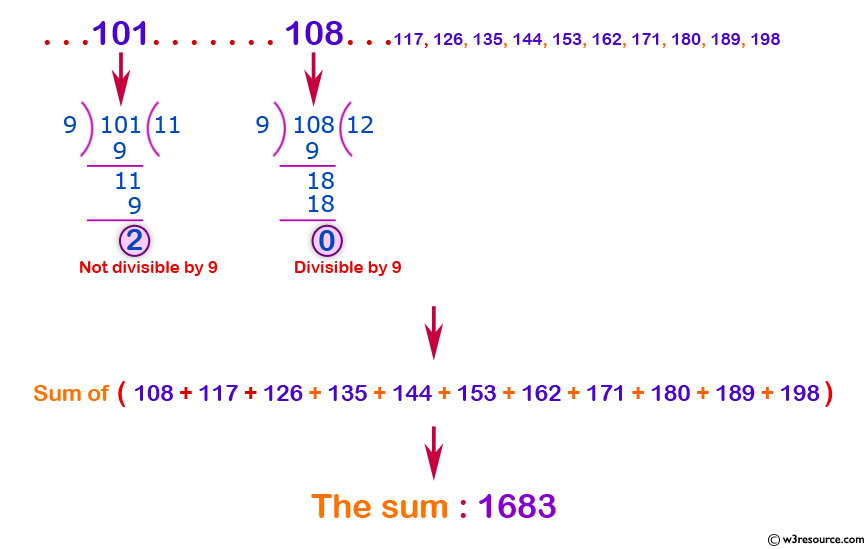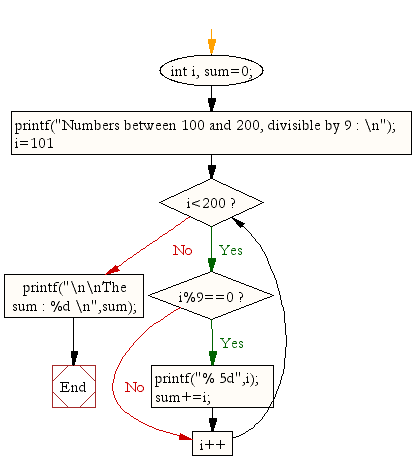﻿ C : Sum of all integers between 100 and 200, divisible by 9# C Exercises: Find the number and sum of all integer between 100 and 200, divisible by 9

## C For Loop: Exercise-39 with Solution

Write a program in C to find the number and sum of all integer between 100 and 200 which are divisible by 9.

Pictorial Presentation:C Code:

``````#include <stdio.h>

void main()
{
int i, sum=0;
printf("Numbers between 100 and 200, divisible by 9 : \n");
for(i=101;i<200;i++)
{
if(i%9==0)
{
printf("% 5d",i);
sum+=i;
}
}
printf("\n\nThe sum : %d \n",sum);
}
```
```

Sample Output:

```Numbers between 100 and 200, divisible by 9 :
108  117  126  135  144  153  162  171  180  189  198

The sum : 1683
```

Flowchart:C Programming Code Editor:

Improve this sample solution and post your code through Disqus.

What is the difficulty level of this exercise?

Test your Programming skills with w3resource's quiz.

﻿

## C Programming: Tips of the Day

You are seeing the ffffff because char is signed on your system. In C, vararg functions such as printf will promote all integers smaller than int to int. Since char is an integer (8-bit signed integer in your case), your chars are being promoted to int via sign-extension.

Since c0 and 80 have a leading 1-bit (and are negative as an 8-bit integer), they are being sign-extended while the others in your sample don't.

```char    int
c0 -> ffffffc0
80 -> ffffff80
61 -> 00000061
```
Here's a solution:
```char ch = 0xC0;
printf("%x", ch & 0xff);
```

This will mask out the upper bits and keep only the lower 8 bits that you want.

Ref : https://bit.ly/3vOLizM